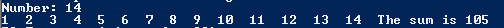# Assignemnt #88

## Code

```///colin hinton
///5th period
///12/4/2015

import java.util.Scanner;

{
public static void main (String [] args)
{
Scanner kb = new Scanner(System.in);

int total = 0;
System.out.print("Number: ");
int num = kb.nextInt();

for (int x = 1; x <= num ;  x = x + 1)
{
total = total + x ;
System.out.print(x + "  ");
}
System.out.println("The sum is " + total);
}
}
```

### Picture of the output